## Why Maple crash when doing String Split on string ...

I have string of length

StringTools:-Length(str);
1073105

When doing

p:=StringTools:-LengthSplit(str,100):

Maple gives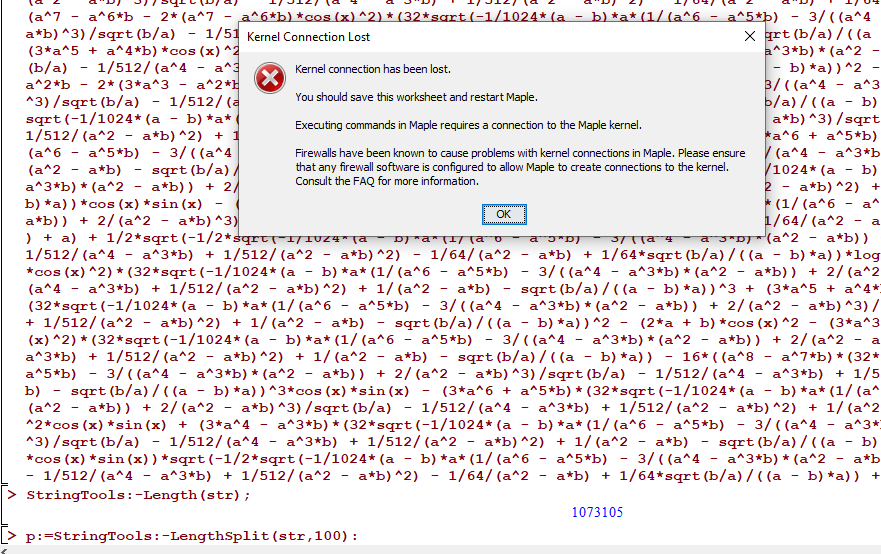From help:

LengthSplit
split a string into equal length pieces

This is all done in .mpl  file actually, not interactive, but I made the above worksheet to show  the problem. The string is read from a file by a program and it wants to split it to 100 length smaller strings.

I have lots of RAM. 128 GB.  So why does Maple crash and is there a workaround this?

This string was generated by another software.

I have attached the above example below as worksheet.  Maple 2022.1 on windows 10.

Here is a simpler way to generate this problem

```restart;
str:=StringTools:-Random(1073104,'upper'):
str[1..10];

StringTools:-Length(str);
p:=StringTools:-LengthSplit(str,100):
```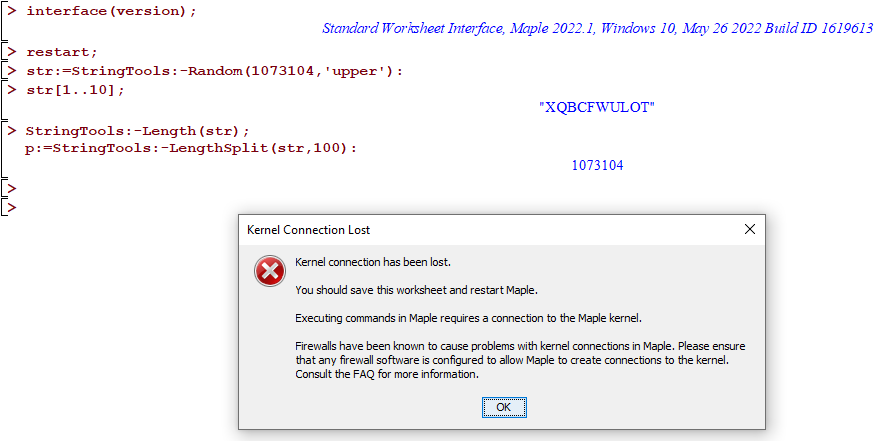Using trial and error, the crash happens when the length reaches 1073101. At 1073100 there was no crash.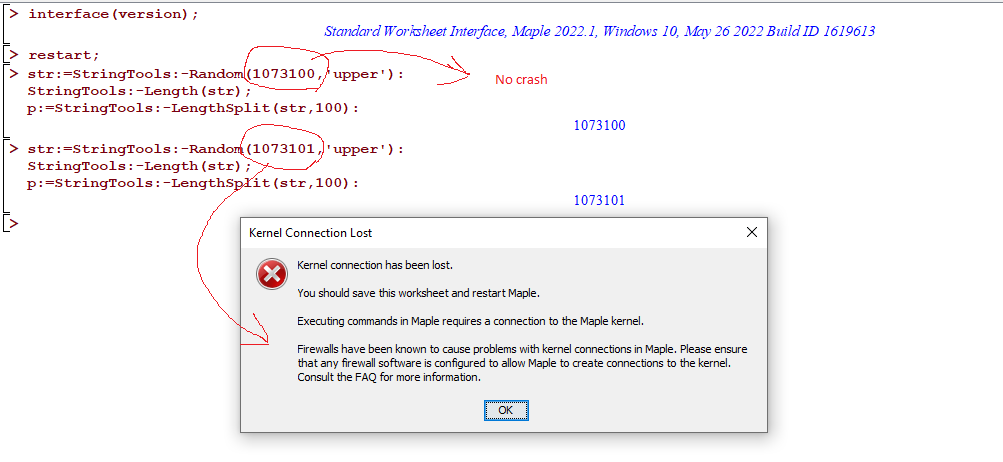## why PDEtools:-Solve gives exception but not solve?...

Found case where PDEtools:-Solve gives exception but not solve.

Is this known issue?

 > interface(version);> restart;
 > result:=1/2*(Dirac(1,-t+4+k)+Dirac(1,t-4+k)-Dirac(1,-t+4+k)*cos(-t+4+k)+2*Dirac(-t+4+k)*sin(-t+4+k)+2*sin(t-4+k)*Dirac(t-4+k)-Dirac(1,t-4+k)*cos(t-4+k))/k; result:=simplify(result) ; PDEtools:-Solve(result = 0,t) assuming t::realError, (in assuming) when calling 'RootOf'. Received: 'expression independent of, _Z, RootOf'

 > solve(result = 0,t) assuming t::real
 >

## Maple in Social Science

Maple 2022

15º Foro de Investigación de las Matemáticas Aplicadas a las Ciencias Sociales "Reflexiones sobre Educación y Matemáticas”,

"Learning of mathematical functions using applications with Maple, for students of Social Sciences"

The students of the first cycles have a low level of learning in the subject of functions, and the arrival of the pandemic worsened the understanding of this content. Increasing the use of ICT in great magnitude improving learning.
To determine the relationship between learning and mathematical functions using applications with Maple, for students of Social Sciences. The experimental method was used using the scientific software Maple applied to students of Social Sciences.

In Spanish.

Lenin Araujo Casillo

## how to read the "originalview" or the range of x a...

I create a plot, then later on, I want to use the same view for a second plot or using the same starting x, ending x and starting y and ending y. For reasons no need to get into into, I need to do this and I have no access to this information at this time. I only have access to the p variable used to store the plot into as in this example below.

I am not sure what is the correct way to do read this information from the PLOTS structure as I never used it before.

Help describes the PLOTS structure under "PLOT and PLOT3D Data Structures" , but do not see how is one supposed to read different parts of it. I could have missed it. Maple help pages are very hard for me to read in order to obtain the information needed. From help it says:

The Maple plotting functions, plot, plot3d, and others, produce PLOT and PLOT3D data structures describing the images to be displayed.

By trial and error, for standard plot this worked

```p:=plot(sin(x),x=-1..10):
op([4,1,2,2],p)
```

returns

"originalview" = [-0.9862500000 .. 9.9862500000, -1.0000000000 .. 1.0000000000]

I see also that command plottools:-getdata(p):  returns the data. So I could also do (it is stored in matrix, in third entry):

```p0:=plottools:-getdata(p);
startingx:=p0[1,1];
startingy:=p0[1,2];
endingx:=p0[-1,1];
endingy:=p0[-1,2];

```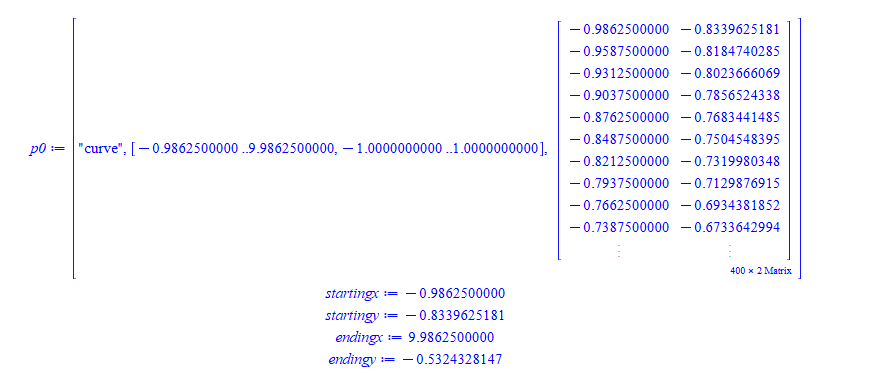Will the above work all the time? should this command be used to obtain this information or is there a better way?

When I lprint(p) I see these fields have actual names. For example  , here is screen shot of lprint(p)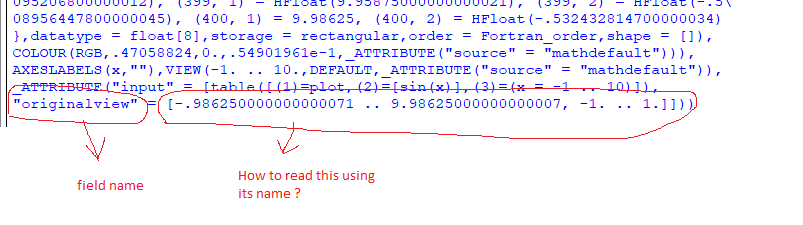it looks like  a RECORD data structure. But when I tried p:-originalview  Error, `p` does not evaluate to a module

The question is: What is the correct way to find what x range and y range was used for an earlier plot, given one has access only to p variable used to store the output of the plot command?  This is for 2D plot only.

## how to set DynamicSystems continuoustimevar to so...

I have differential equation with x as independent variable that I want to convert to state space representation.

But DynamicSystems will not accept y(x) as the output variable, since it defaults to y(t).

Help under "Description of the Model of a Linear System Object" says it is possible to change the independent variable by changing continuoustimevar setting. But help does not give an example how to do this and all my tries failed.

How to do this? Attached is worksheet showing the problem

 > interface(version);> restart; ode:=diff(y(x),x\$2)+y(x) = 0; DynamicSystems:-DiffEquation(ode,'outputvariable'=[y(x)]);Error, (in DynamicSystems:-DiffEquation) missing output(s): {y(t)}

 > restart; ode := diff(y(t),t\$2) + y(t) =0; sys:=DynamicSystems:-DiffEquation(ode,'outputvariable'=[y(t)]);## why DEplot behave differently when using different...

Using t=0 .. 3*Pi the plot is truncated. Changing the 3*Pi to a number, then the full plot shows.

Also keeping 3*Pi but changing y=-1..1 to y=-1.01 .. 1.01 now the full plot shows again.

Is the above normal behaviour or it it a bug?

 > interface(version);> restart; ode := diff(y(t), t\$2) + y(t)=0; DEtools:-DEplot(ode, y(t), t=0 .. 3*Pi, y=-1 .. 1,[[y(0)=1,D(y)(0)=0]],linecolor=blue);> #replacing 3*Pi by a number, then it shows the full plot restart; ode := diff(y(t), t\$2) + y(t)=0; DEtools:-DEplot(ode, y(t), t=0 .. 10, y=-1 .. 1,[[y(0)=1,D(y)(0)=0]],linecolor=blue);> #keeping 3*Pi but changing the y range, it now also show the full plot restart; ode := diff(y(t), t\$2) + y(t)=0; DEtools:-DEplot(ode, y(t), t=0 .. 3*Pi, y=-1.01 .. 1.01,[[y(0)=1,D(y)(0)=0]],linecolor=blue);>

## How can an equation be sorted by numerical value o...

I want to collect up the equation terms by the numerical value of the terms coefficient? Have tried sort collect combine...
So far the best I have come up with is nops(indets(on each term). And put them in seperate lists. This still doesn't quiet do the trick.
I am looking to achieve. Would to happy to have then as seperate lists or equations.

`(a_1^5+a_2^5...)+5(a_1^4a_2+a_1^4a_3....)+10(a_1^3a_2^2 ....)+20(  ....   )+......+60(a_1^2a_2a_3a_4+ a_1a_2^2a_3a_4....)`## Galerkin method...

I am dealing with a system of differential equations and this method is galerkin method i just cant understand why it is not working for positive Rn parameter value.>(1)
 >(2)
 >(3)
 >(4)
 >>>>>>>>>> for i from 1 by 1 to n do;
 > for j from 1 by 1 to n do;
 > M1[i,j]:=F1(i,j);M2[i,j]:=F2(i,j);M3[i,j]:=F3(i,j);;M1;M2;M3;
 > M4[i,j]:=F4(i,j);M5[i,j]:=F5(i,j);M6[i,j]:=F6(i,j);;M4;M5;M6;
 > M7[i,j]:=F7(i,j);M8[i,j]:=F8(i,j);M9[i,j]:=F9(i,j);;M7;M8;M9;
 > end do;
 > end do;
 >
 >
 > M[1 .. n, 1 .. n] := M1 : M[1 .. n, n+1 .. 2*n] := M2 :  M[1 .. n, 2*n+1 .. 3*n] := M3:
 > M[n+1 .. 2*n, 1 .. n] := M4  :  M[n+1 .. 2*n, n+1 .. 2*n] := M5 : M[n+1 .. 2*n, 2*n+1 .. 3*n] := M6 :
 > M[2*n+1 .. 3*n, 1 .. n] := M7 : M[2*n+1 .. 3*n, n+1 .. 2*n] := M8  :  M[2*n+1 .. 3*n, 2*n+1 .. 3*n] := M9:
 >
 >>>>> T1:=[];T2:=[];
 > for Q from 0 by 20 to 100 do;
 > eq := LinearAlgebra:-Determinant(M)= 0;
 > m:=solve(eq, R):
 > mi:=Optimization[Minimize](m, k = 0 .. 7);
 > T1:=[op(T1),[Q,rhs(mi)]];
 > T2:=[op(T2),[Q,mi]];
 > end do:
 > p1:=evalm(T1);
 > p2:=evalm(T2);
 > writedata("p1-Peinf-x00001.txt", p1, float);
 > writedata("p2-Peinf-x00001.txt", p2, float);
 >(5)
 >>>>>>>I just want to plot when Rn value is non-zero.

## How to calculate the fractional derivative of an ...

Dear All,

I have a question about "fraddiff" command to compute the fractional derivative of a function.
I need to produce  a procedure to manually compute Caputo fractional derivative of a function, instead of using "fracdiff", as the following:

Frac_C:=proc()
description "Frac_C(function,lower bound, variable (upper bound), order of differentiation)";
a:=args;
x:=args;
alpha:=args;
f:=unapply(args,args);
m:=ceil(alpha);
return 1/GAMMA(m-alpha)*int((x-tau)^(m-alpha-1)*diff(f(tau),tau\$m),tau=a..x);
end proc;

when I call the above procedure, as Frac_C(x^(3.4),0,x,3/4), Maple is not able to calculate the integral defined in the Caputo fractional derivative. However, I saw the pre-defined Maple command, namely fracdiff, is able to do.
Where is the trouble?
can anyone help me?

It is worth mentioning that I can calculate the fractional derivative of a functional by procedure "Frac_C()" when I try to use it at the given x as the following which is not desirable for me.
evalf(subs(x=2,Frac_C(x^(3.4),0,x,3/4)));
evalf(subs(x=2,fracdiff(x^(3.4),x,3/4)));

Best wishes

## y(x) is the result of the last calculation. How do...

Download a.mwThe question is in the picture.

y(x) is the result of the last calculation. How do I apply diff to the last result?

Thanks!

## Should combine here have automatically combined te...

Given two terms in expression of type  `+` where the first is rational and the second is not, I wanted to combine both under on common denominator to obtain one term (not `+` any more).

I expected that combine will do that.

```restart;
expr:=A/B+C;
combine(expr);
```

But the above leaves the original expression as is. Ofcourse I can just do

```numer(expr)/denom(expr);
```

Here is screen shot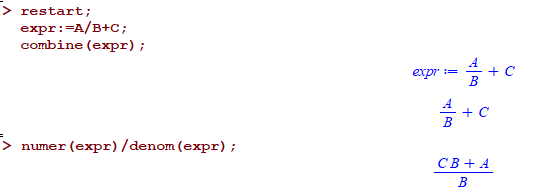My question is why combine does not do that automatically? For reference, Mathematica command Together is the equivalent to Maple combine and this is what it does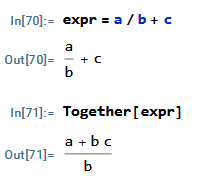Which is what one would expect to happen.

I am just trying to understand the logic why Maple's combine did not work as one would expect. That is all. I looked at help and tried different option to combine, but non worked.

## Physics[Vectors] vector type...

Is there  a specific type name for vectors in Physics[Vectors]?  Specifically, Let's say we want to write a proc whose argument is expected to be a (Physics) Vector, as in  these (trivial) demos:

```with(Physics[Vectors]);

f := proc(a_::???)
return a_ . a_;
end proc:

g := proc(a_::???, b_::???)
return a_ &x b_;
end proc:
```

What do we put in place of "???".